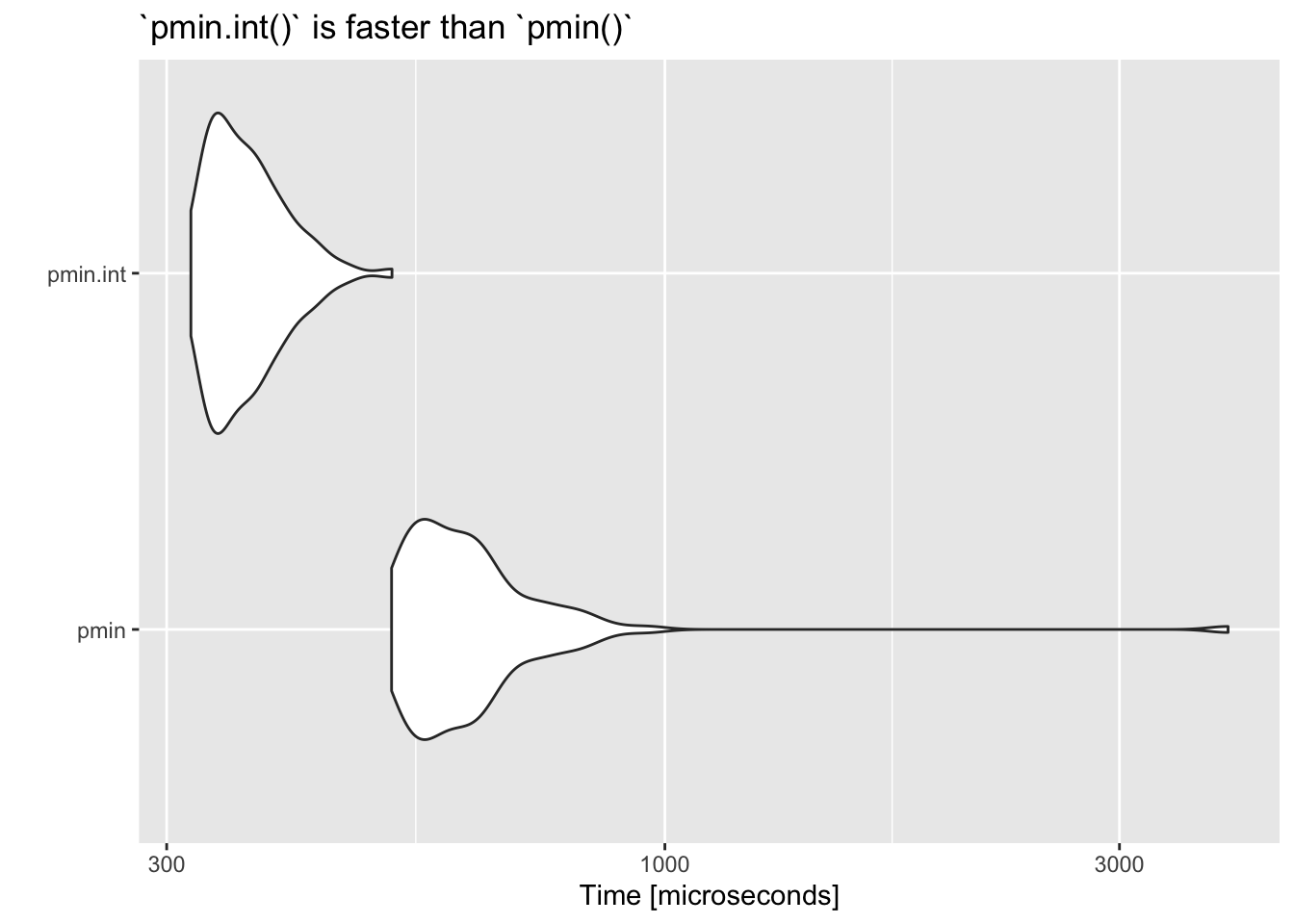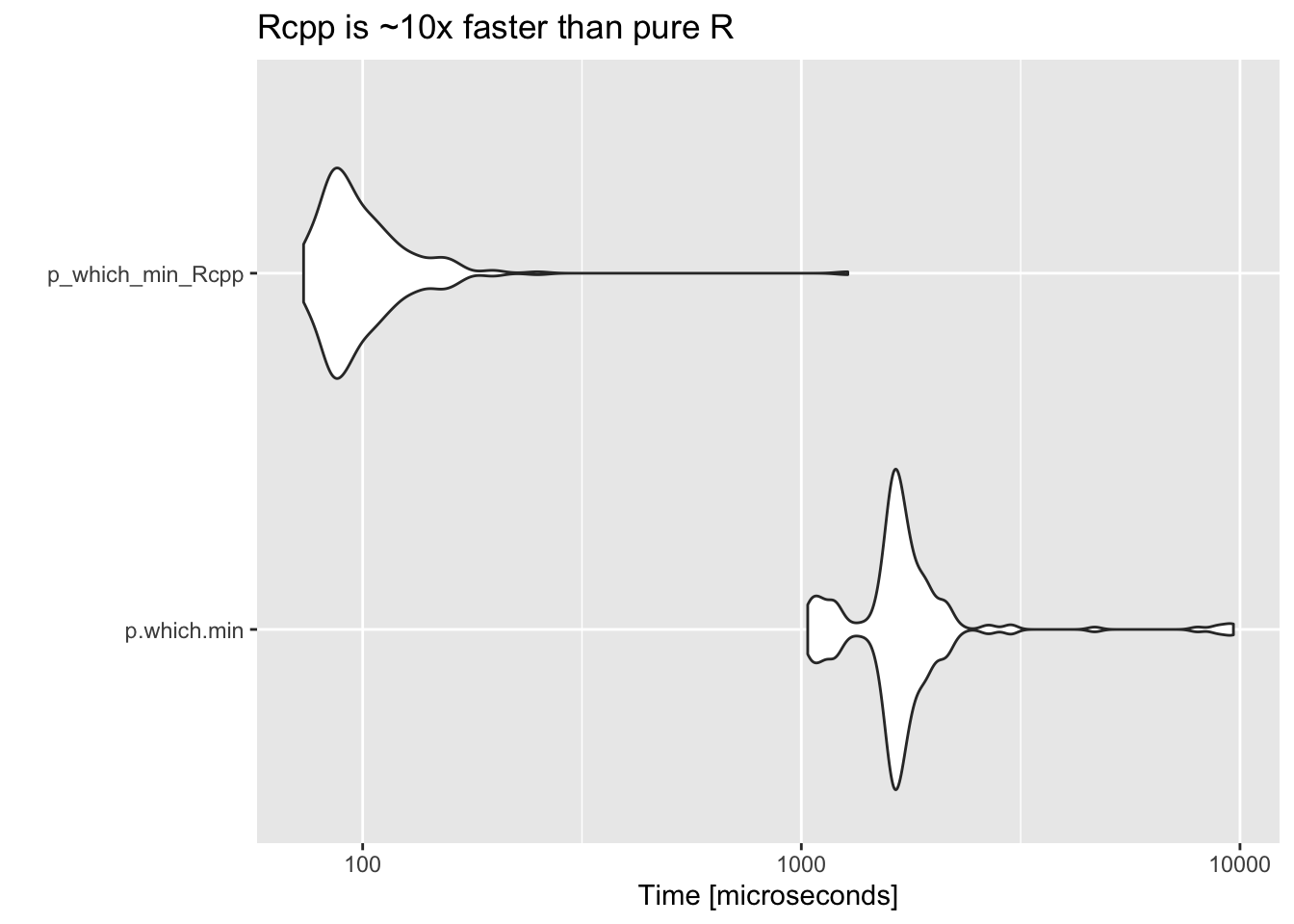# Challenge: Simultaneous parallel min() and which.min()

## Problem: p.which.min()

• Input: vs A list of N vectors of numeric values (each the same length, L)
• Output: For each index along the vectors (1..L)
• the minimum value out of the N vectors at this index
• the index of the vector that had the minimum (1..N)
• Conditions:
• If there are multiple vectors with the same minimum at the given index, it doesn’t matter which is returned.
• The values are floating points (not integers).

This problem is a combination of:

* find the parallel min, and
* find the parallel which.min

.. and so it shall be named p.which.min() !!

## Preamble

Some R functions discussed below operate on lists of vectors, others operator on matrixes. The following 2 functions switch between the two data representations.

vs_to_mat <- function(vs) { do.call(cbind, vs) }
mat_to_vs <- function(mat) {lapply(seq_len(ncol(mat)), function(i) mat[,i])}

## Example

• An example with N=3, L=5
vs <- list(
c(3, 4, 6, 9, 2),
c(9, 9, 7, 6, 1),
c(2, 2, 7, 4, 8)
)

mat <- vs_to_mat(vs)
mat
     [,1] [,2] [,3]
[1,]    3    9    2
[2,]    4    9    2
[3,]    6    7    7
[4,]    9    6    4
[5,]    2    1    8

In this example, the parallel minimum across all vectors is c(2, 2, 6, 4, 1) and the index of the vector in which the minimum occurs is c(3, 3, 1, 3, 2)

# desired result
list(
min   = c(2, 2, 6, 4, 1),
index = c(3, 3, 1, 3, 2)
)

## Create test data

• This is the function used to create benchmarking data, e.g. create_test_data(N=100, L=100)
#-----------------------------------------------------------------------------
# Create a list of N numeric vectors, each with L elements
#-----------------------------------------------------------------------------
create_test_data <- function(N, L) {
set.seed(1)
seq(N) %>% purrr::map(~runif(L))
}

#-----------------------------------------------------------------------------
# Benchmarking data
#-----------------------------------------------------------------------------
vs.bench  <- create_test_data(300, 300)
mat.bench <- vs_to_mat(vs.bench)

## Find the parallel minimum: The pmin() function

The pmin function is part of base R and are exactly what is needed for the first part of this problem.

pmin returns the parallel minima of the input values. That is, it will return the value of the minimum at each index (but not the index of the vector from which it came)

pmin takes a ... argument, so either pass in all the vectors one-at-a-time in the function call or wrap it with a do.call() i.e. do.call(pmin.int, vs)

pmin(c(3, 4, 6, 9, 2), c(9, 9, 7, 6, 1), c(2, 2, 7, 4, 8))
 2 2 6 4 1
do.call(pmin, vs)
 2 2 6 4 1

There is also a built-in pmin.int. The int suffix stands for internal, not integer! This is a faster version of the pmin function when all arguments are atomic vectors and there are no classes.

do.call(pmin.int, vs)
 2 2 6 4 1## Find the parallel which.min: The max.col function

The max.col(matrix) function finds the maximum position for each row of a matrix. For some reason there is no corresponding min.col, but this can be faked with max.col(-matrix).

This only operates on a matrix, so the vs list will need to be converted to a matrix

mat <- vs_to_mat(vs)
max.col(-mat)
 3 3 1 3 2

## R solution - using pmin.int and max.col

#-----------------------------------------------------------------------------
# Find parallel min and which.min over a list of vectors
#-----------------------------------------------------------------------------
p.which.min <- function(vs) {
mins  <- do.call(pmin.int, vs)
mat   <- vs_to_mat(vs)
index <- max.col(-mat)
list(
min   = mins,
index = index
)
}

p.which.min(vs)
$min  2 2 6 4 1$index
 3 3 1 3 2

## Naive Rcpp code

• Initial Rcpp code for
• Anyone have a more idiomatic approach?
Rcpp::cppFunction('
List p_which_min_Rcpp(List vs) {

int N           = vs.size();
NumericVector v = vs;
int L           = v.size();

NumericVector min_so_far(L);
IntegerVector min_index(L);  // Automatically initialised to zero

// Initialise the "min so far" to just be the first vector
min_so_far = v;

// Iterate over each vector in turn (starting from the second one)
for (int i=1; i<N; i++) {
NumericVector vi = vs[i];
for (int j=0; j<L; j++) {
if (vi[j] < min_so_far[j]) {
min_so_far[j] = vi[j];
min_index[j]  = i;
}
}
}

List ret;
ret["min"]   = min_so_far;
ret["index"] = min_index + 1;  // convert to R based indexing
return ret;
}')

p_which_min_Rcpp(vs)
$min  2 2 6 4 1$index
 3 3 1 3 2

## Benchmarking

Unit: microseconds
expr      min       lq      mean    median        uq      max
p.which.min 1034.128 1558.653 1850.7818 1651.3805 1814.9050 9661.718
p_which_min_Rcpp   73.336   84.684  106.9455   93.4695  108.6985 1276.364
neval
200
200## Conclusions and Extensions

• Anyone know of a better pure R solution?
• Would still like an idiomatic Rcpp version.